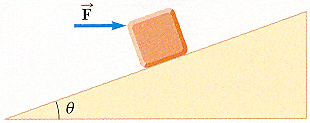# Block on a ramp! Sum of forces, normal force, static friction, etc! Question!

Ok so I already know how to solve it by looking at an example my teacher did in class...
This is what I did...

4. A 2.00 kg block is held in equilibrium on an incline of angle θ = 70° by a horizontal force vector F applied in the direction shown in the figure below. If the coefficient of static friction between block and incline is μs = 0.300, determine the following.(a) the minimum value of F

Step 1) Draw Free Body Diagram
Step 2) Determine forces in x and y direction

Fx
fs
F cos 70
-mg sin 70

Fy
n
-F sin 70
-mg cos 70

Step 3) Sum of x-forces and sum of y-forces/Equilibrium equations

ƩFx = fs + F cos 70 + (-mg sin 70) = 0

ƩFy = n + (-F sin 70) + (-mg cos 70) = 0
ƩFy = n + (-F sin 70) + (-mg cos 70) = 0
ƩFy = n - F sin 70 - mg cos 70 = 0
ƩFy = n = F sin 70 + mg cos 70
ƩFy = n = 0.940F + (2.00)(9.8)cos 70
ƩFy = n = 0.940F + 6.704

Step 4) Solve for static friction fs

fs = μsn
fs = (0.300)n
fs = (0.300)(0.940F + 6.704)
fs = 0.282F + 2.0112

Step 5) Find the HORIZONTAL force vector by plugging in fs in ƩFx

ƩFx = fs + F cos 70 + (-mg sin 70) = 0
ƩFx = (0.282F + 2.0112) + 0.342F - 18.418 = 0
ƩFx = 0.282F + 0.342F + 2.0112 - 18.418 = 0
ƩFx = 0.624F - 16.4068 = 0
ƩFx = 0.624F = 16.4068
ƩFx = F = 26.29 N

(b) the normal force exerted by the incline on the block

n = 0.940F + 6.704
n = 0.940(26.29) + 6.704
n = 31.4 N

MY QUESTION IS...

In STEP 2, why is the force "-mg sin 70" included in the X-DIRECTION and why is the force -mg cos 70 included in the Y-DIRECTION????

I thought the ONLY forces acting in the x-direction were static friction, fs and Fcos 70, while the only forces acting in the y direction were normal force, n, and -Fsin 70???

Help!

In STEP 2, why is the force "-mg sin 70" included in the X-DIRECTION and why is the force -mg cos 70 included in the Y-DIRECTION????

I thought the ONLY forces acting in the x-direction were static friction, fs and Fcos 70, while the only forces acting in the y direction were normal force, n, and -Fsin 70???

Help!
----------------------------------
There are 4 forces acting on the block, weight ,applied force,frictional force and normal.
We create a new frame of reference with x-axis parallel to the inclined plane
So you have to resolved all the forces to new x and y direction for easy arithmetic.

The frictional force is parallel to the plane and opposes the motion. Without friction the block will slide down. So the friction is opposite direction to that.

The normal force is acting in y direction which is equal to μN

Draw fbd or vector diagram on these 4 forces with their components.

Last edited:
In STEP 2, why is the force "-mg sin 70" included in the X-DIRECTION and why is the force -mg cos 70 included in the Y-DIRECTION????

I thought the ONLY forces acting in the x-direction were static friction, fs and Fcos 70, while the only forces acting in the y direction were normal force, n, and -Fsin 70???

Help!
----------------------------------
There are 2 forces acting on the block, weight and applied force.
We create a new frame of reference with x-axis parallel to the inclined plane
So you have to resolved the 2 forces to new x and y direction for easy arithmetic.

So basically... whenever I have a problem like this, where I have Fcos 70 as a force in the x direction, the opposite(-Fsin 70) would also have to be taken into account and vice versa?

So basically... whenever I have a problem like this, where I have Fcos 70 as a force in the x direction, the opposite(-Fsin 70) would also have to be taken into account and vice versa?

Sorry for so many editing on my last post.

The best thing to do is to draw a vector diagram(for a start, many diagrams).
1. First draw vector diagram with force applied and the weight.
2. Replace those 2 forces with their components in x an y direction.
3. You add negative y direction forces. This value is opposed by equal Normal force.
4. Then frictional force emerge due to Normal force formed and the coefficient not equal to zero.

Hope you can post your diagram.
Take a snap, and use imageshack.com or other sites to upload the diagram and copy the link.

Last edited: